# Natural transformation in differential geometry

(diff) ← Older revision | Latest revision (diff) | Newer revision → (diff)

The classical theory of differential-geometric objects was revisited from the functorial point of view by A. Nijenhuis, [a3]. He defined a natural bundleover-dimensional manifolds as a functor transforming every-dimensional manifoldinto a fibred manifold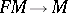over(cf. Fibred space) and every local diffeomorphism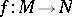into a fibred manifold morphism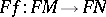over. Later it was taken into consideration that certain geometric objects can be constructed on certain special types of manifolds only. This led to an analogous concept of bundle functor on a category over manifolds, [a1].

From this point of view, a geometric construction on the elements of one bundle of a functorwith values in the bundle of another functorover the same base has the form of a natural transformation. Moreover, theth order natural operators ofinto(cf. Natural operator in differential geometry) are in bijection with the natural transformations of the bundle functor of theth jet prolongationinto.

In the simplest case, ifandare twoth order natural bundles over-dimensional manifolds, the natural transformationsare in bijection with the-equivariant mappings between their standard fibres, where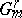is the jet group of orderin dimension. Several methods for finding-equivariant mappings in the-case are collected in [a1]. If manifolds with an additional structure are studied, one has to consider the corresponding subgroup of.

Many problems on finding natural transformations between geometrically interesting pairs of bundle functors are solved in [a1]. Even a negative answer can be of geometric interest. For example, in [a1] it is deduced that there is no natural equivalence between the iterated tangent functor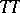and the composition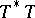of the cotangent and the tangent functors. This implies that, unlike for the cotangent bundle, there is no natural symplectic structure on the tangent bundle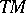of a manifold.

The complete description of all natural transformations between two product-preserving bundle functorsandon the category of all manifolds and all-mappings was deduced in the framework of the theory of bundle functors determined by local algebras, which was established by A. Weil, [a4] (cf. also Weil algebra). Eachorcorresponds to a local algebraor, respectively, and all natural transformationsare in bijection with the algebra homomorphisms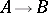, see [a1] for a survey. An analogous characterization of all natural transformations between two product preserving bundle functors on the category of fibred manifolds was deduced by W. Mikulski, [a2].

How to Cite This Entry:
Natural transformation in differential geometry. Encyclopedia of Mathematics. URL: http://encyclopediaofmath.org/index.php?title=Natural_transformation_in_differential_geometry&oldid=13906
This article was adapted from an original article by Ivan KolÃ¡Å™ (originator), which appeared in Encyclopedia of Mathematics - ISBN 1402006098. See original article